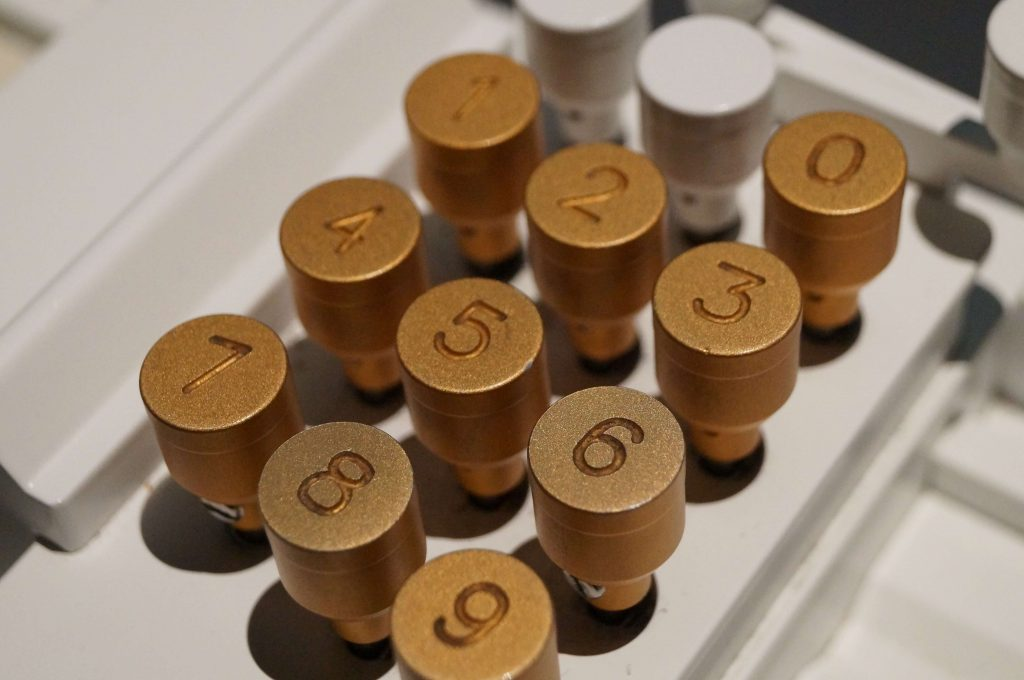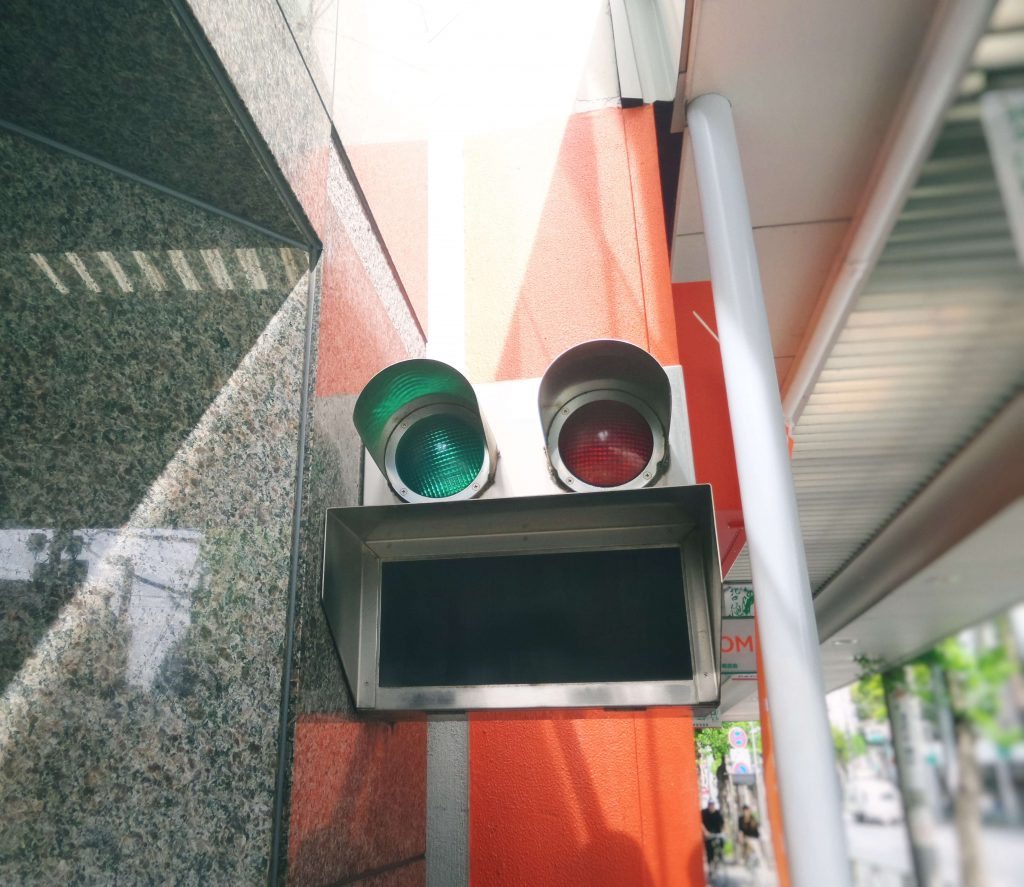Got Feedback?

Found a bug? Have a suggestion? Fill the form below and we'll take a look!

X

# Understanding the “inside out” of number system: (Part 1)

## Number System Conversions:

The number system, which we have discussed in the previous article named “let’s operate the number system”. In which we talk about the relationship of (Decimal, Binary, Octal, and Hexadecimal). We can convert a number of a particular system to its equivalent number in other systems.

## Conversion from Decimal system to other systems:

The method of converting from decimal to another system is by successive division by the base of the objective system. The remainders of these divisions are arranged in reverse order.

## Decimal to Binary Conversions:

To convert from decimal to binary, divide the decimal number by 2, which is the base of the binary system.

Here we have decimal 57 and going to convert into its binary equivalent.

The binary number is obtained by arranging the remainders from bottom to top. Thus, the equivalent binary number is : (111001)

## Decimal to Octal Conversion:

To convert a decimal number to its octal equivalent, divide the decimal number successively by 8 in a similar manner as was done with decimal to binary conversions. The remainders are read in reverse order to obtain the required octal number.

Here we have decimal digits 1769 and going to convert into its octal to equivalent:

Thus, we got octal number which is (3351).

## Decimal to Hexadecimal conversions:

Decimal to Hexadecimal conversions can be carried out using the same technique as shown above binary and octal conversion with the division being done by 16.

Here we have decimal digits 6958 and going to convert into its hexadecimal equivalent.

Here, you need to know the in hexadecimal number system that alphabet

• A denotes 10
• B denotes 11
• C denotes 12
• D denotes 13
• E denotes 14
• F denotes 15
• G denotes 16

Therefore the equivalent of this decimal number in hexadecimal is (1B2E).

## Let’s learn Conversion from other systems to the Decimal system:

Converting from other systems to decimal system involves multiplication.

Multiply each digit of the given number by its positional weight. The base of the given number multiplies each positional weight.

Final value is obtained by adding each positional value.

We can perform this process through these steps.

Step 1: Multiply each digit of the given  number by its base raised to positional power.

Step 2: Add all results of multiplication of each position.

## Binary to Decimal Conversion:

We convert binary to decimal by finding the decimal equivalent of the binary array of digits (10011)2 and expanding the binary digits into a series with a base of 2 giving an equivalent of (19)10 in decimal or denary.

Note that in number conversion systems “subscripts” are used to indicate the relevant base numbering system, 10012 = 910. If no subscript is used after a number, then it is generally assumed to be decimal.

Let’s convert decimal number to binary (10011)2:

= 1 x 24 + 0 x 23 + 0 x 22 + 1 x 21 + 1 + 20

= 1 x 16 + 0 x 8 + 0 x 4 + 1 x 2 + 1 x 1

= 16 + 0 + 0 + 2 + 1

= ( 19 )10

The equivalent decimal number is 19 for binary number 10011.

When converting from Binary to Decimal or even from Decimal to Binary, we need to be careful that we do not mix up the two sets of numbers.

For example, if we write the digits 10 on the page it could mean the number “ten” if we assume it to be a decimal number, or it could equally be a “1” and a “0” together in binary, which is equal to the number two in the weighted decimal format from above. for example, if we were using a binary number string we would add the subscript “2” to denote a base-2 number so the number would be written as 102. Likewise, if it was a standard decimal number we would add the subscript “10” to denote a base-10 number so the number would be written as 1010.

## Octal to Decimal Conversion:

Octal numbers, therefore, have a range of just “8” digits, (0, 1, 2, 3, 4, 5, 6, 7) making them a Base-8 numbering system and therefore, q is equal to “8”.

Convert the octal number (546)8 to its decimal number equivalent, (base-8 to base-10).

Step 1: = 5 x 82 + 4 x 81 + 6 x 80

= 5 x 64 + 4 x 8 + 6 x 1

Step 2: = 320 + 32 + 6

= (358)10

Converting octal to decimal shows that (546)8 in its Octal form is equivalent to (358)10 in its Decimal form.

While Octal is another type of digital numbering system, it is little used these days instead of the more commonly used Hexadecimal Numbering System is used as it is more flexible.

## Hexadecimal to Decimal Conversions:

The “Hexadecimal” or simply “Hex” numbering system uses the Base of 16 system and is a popular choice for representing long binary values because their format is quite compact and much easier to understand.

Then in the Hexadecimal Numbering System, we use the numbers from 0 to 9 and the capital letters A to F to represent it’s Binary or Decimal number equivalent, starting with the least significant digit at the right-hand side.

Convert the following Hexadecimal number (3AC8)16 into Decimal or Denary equivalent using subscripts to identify each numbering system.

Step 1: = 3 x 163 + A x 162 + C x 161 + 8 x 160

= 3 x 4096 + 10 x 256 + 12 x 16 + 8 x 1

Step 2 = 12288 + 2560 + 192 + 8

= (15048)10

Then, the Decimal number of 15048 can be represented as 3AC8 in Hexadecimal.

# How to understand Numbers System in an easy way?

The number system is the system of counting and calculations. Number system is formed of  characters called “Digits”. Each number is made up of these characters. The number of digits a system uses is called its base or Radix. For example the number system we use in our habitual life is called Decimal System.Photo by James Orr on Unsplash

There are four branches of the number system.

1) Decimal    2) Binary   3) Octal   4) Hexadecimal

## Decimal system:

The number system which we use in our habitual life is based on ten digits. 0, 1, 2, 3, 4, 5, 6, 7, 8, and 9. Any numbers can be expressed as a combination of these ten digits. For example, 25 is the combination of two digits 2 and 5, 125 is the combination of three digits and so on. In this system, every digit of a number is a multiple of some power of 10.

Any decimal integer can be expressed as the sum of each of its digits times power of 10.

Since this number system has ten digits, this system is also called “Decimal Number System” Or “ Denary System” or  “ Base ten system”.

For example, 8326 can be expressed as:

= 8 x 103 + 2 x 102 + 3 x 101 + 6 x 100

= 8 x 1000 + 2 x 100 + 3 x 10 + 6 x 1

= 8000 + 200 + 30 + 6

Position Weight

1st 100= 1
2nd 101= 10
3rd 102= 100
4th 103= 1000
5th 104 =10000

## Binary System:Photo by Franck V. on Unsplash

The binary number system is ideal for internal networking of electronic computers.

The Binary System is especially useful in the construction of computers because the digits can be represented by switches in “ON” or “OFF” positions.Computer circuitry represents data in a pattern of ON or OFF state of electric current. Because there are only two states, they are represented by 1 (for ON) and 0( for OFF). Binary system is based on two fundamental digits 0 and 1 to represent its numeric values there the base of this system is two.So, it is also called “Base two number system”.

The positional weight for the binary system are based on the powers of two.To visualized the positional values of the binary system the weights for the first five positions are given as under:

1st 20= 1
2nd 21= 2
3rd 22= 4
4th 23= 8
5th 24 =16

Here, we have an example 100112 can be represented in decimal as:

=1 x 24 + 0 x 23 + 0 x 22 + 1 x 21 + 1 x 20

= 1 x 16 + 0 x 8 + 0 * 4 + 1 x 2 + 1 x 1

= 16 + 0 + 0 + 2 + 1

= 19

## The octal system:

Octal is latin word for eight (8). Octal has eight fundamental digits as, 0, 1, 2, 3, 4, 5, 6, and 7. So, the base of this system is eight. The weights of digits position are successive powers of eight as under :

Position Weight

1st 80= 1
2nd 81= 8
3rd 82= 64
4th 83= 512
5th 84 = 4096

Here, we have an example 3528 can be expressed in decimal as:

= 3 x 8 2 + 5 x 8 1 + 2 x 8 0

= 3 x 64 + 5 x 8 + 2 x 1

= 192 +40 + 2

= 234 10

## Hexadecimal system:

Hexadecimal means 16, therefore this is a base -16 number system.It has 16 basic digits. The first ten digits are same as decimal (0 to 9) and the rest of the six digits are the first six letters of english alphabet (a, b, c, d, e, f).The letters A through F represent the decimal numbers 10 through 15.This system is often used in programming as a shortcut to the binary number system. The weight used in the hexadecimal system are the successive power of 16.

The weight of the first five digits positions are as under.

Position Weight

1st 160 = 1
2nd 161 = 16
3rd 162 = 256
4th 163 = 4096
5th 164 = 65536

Here, we have an example 3AC8 can be expressed in decimal as:

= 3 x 16 3 + A x 16 2 + C x 16 1 + 8 x 16 0

= 3 x 4096 + 10 x 256 + 12 x 16 + 8 x 1

= 12288 + 2560 + 192 + 8

= 15048 10